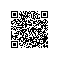# 记一道有意思的算法题Rotate Image（旋转图像）

You are given an n x n 2D matrix representing an image.

Rotate the image by 90 degrees (clockwise).

Could you do this in-place?

a b c d

c a d b

a=a^b; b=a^b; a=a^b;

a = a ^ b ^ c ^ d; b = a ^ b ^ c ^ d; d = a ^ b ^ c ^ d; c = a ^ b ^ c ^ d; a = a ^ b ^ c ^ d;

public void Rotate(int[,] matrix) { int n = matrix.GetLength (0); for (var i = 0; i < (n + 1)/2; i++) { for (var j = 0; j < n/2; j++) { //var a = matrix[i, j]; //var b = matrix[j, n - i - 1]; //var d = matrix[n - i - 1, n - j - 1]; //var c = matrix[n - j - 1, i]; matrix[i, j] = matrix[i, j] ^ matrix[j, n - i - 1] ^ matrix[n - i - 1, n - j - 1] ^ matrix[n - j - 1, i]; matrix[j, n - i - 1] = matrix[i, j] ^ matrix[j, n - i - 1] ^ matrix[n - i - 1, n - j - 1] ^ matrix[n - j - 1, i]; matrix[n - i - 1, n - j - 1] = matrix[i, j] ^ matrix[j, n - i - 1] ^ matrix[n - i - 1, n - j - 1] ^ matrix[n - j - 1, i]; matrix[n - j - 1, i] = matrix[i, j] ^ matrix[j, n - i - 1] ^ matrix[n - i - 1, n - j - 1] ^ matrix[n - j - 1, i]; matrix[i, j] = matrix[i, j] ^ matrix[j, n - i - 1] ^ matrix[n - i - 1, n - j - 1] ^ matrix[n - j - 1, i]; } } }

a=a+b; b=a-b; a=a-b;使用钉钉扫一扫加入圈子
+ 订阅